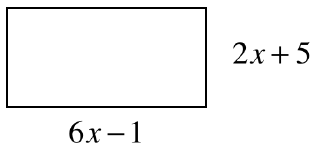### Home > GC > Chapter 1 > Lesson 1.1.3 > Problem1-25

1-25.

Read the Math Notes box for this lesson, which describes how to find the area and perimeter of a shape. Then examine the rectangle at right. If the perimeter of this shape is $120$ cm, which equation below represents this fact? Once you have selected the appropriate equation, solve for $x$. Homework Help ✎1. $2x+5+6x−1=120$

1. $4(6x−1)=120$

1. $2(6x−1)+2(2x+5)=120$

1. $(2x+5)(6x−1)=120$

• The equation for the perimeter is part (c).

$2(6x−1)+2(2x+5)=120$
$(12x−2)+(4x+10)=120$
$\ \ \ \ \ \ \ \ \ \ \ \ \ \ \ \ \ \ \ \ \ \ \ \ 16x+8=120$
$\ \ \ \ \ \ \ \ \ \ \ \ \ \ \ \ \ \ \ \ \ \ \ \ \ \ \ \ \ \ 16x=112$
$\ \ \ \ \ \ \ \ \ \ \ \ \ \ \ \ \ \ \ \ \ \ \ \ \ \ \ \ \ \ \ \ \ \ x=7$